# RS Aggarwal Solutions for Class 6 Chapter 3 Whole Numbers Exercise 3E

RS Aggarwal Solutions for Class 6, Chapter 3, Whole Numbers plays a vital role in students’ lives for the exam preparation. Solutions are given here with the free download option. Exercise 3E of RS Aggarwal Solutions for Class 6 are created by experts of the subject with the aim of making the concepts clear among students. This exercise has questions related to division in Whole Numbers and properties of division. RS Aggarwal Solutions are designed according to the current syllabus to help students to score well in the exam.

## Download PDF of RS Aggarwal Solutions for Class 6 Chapter 3 Whole Numbers Exercise 3E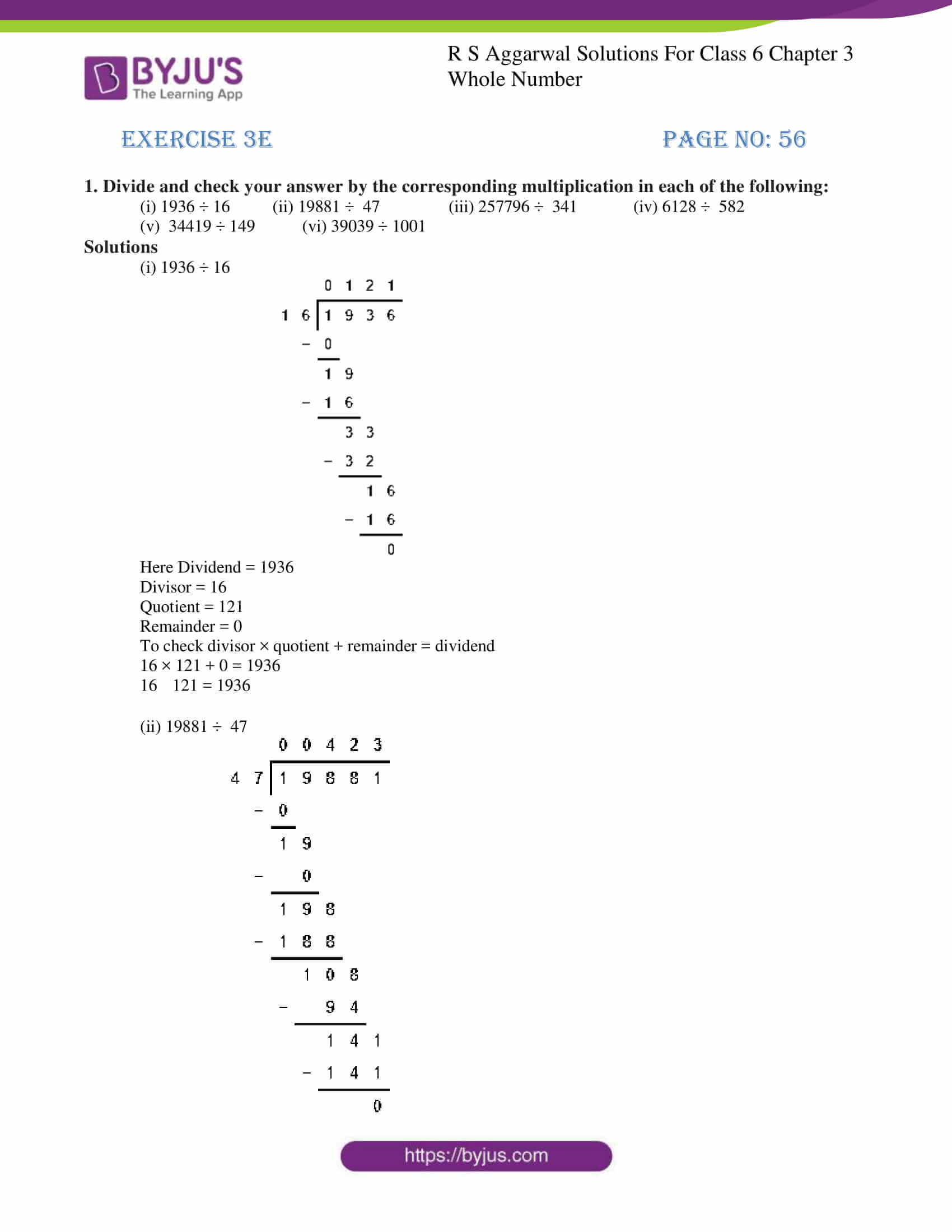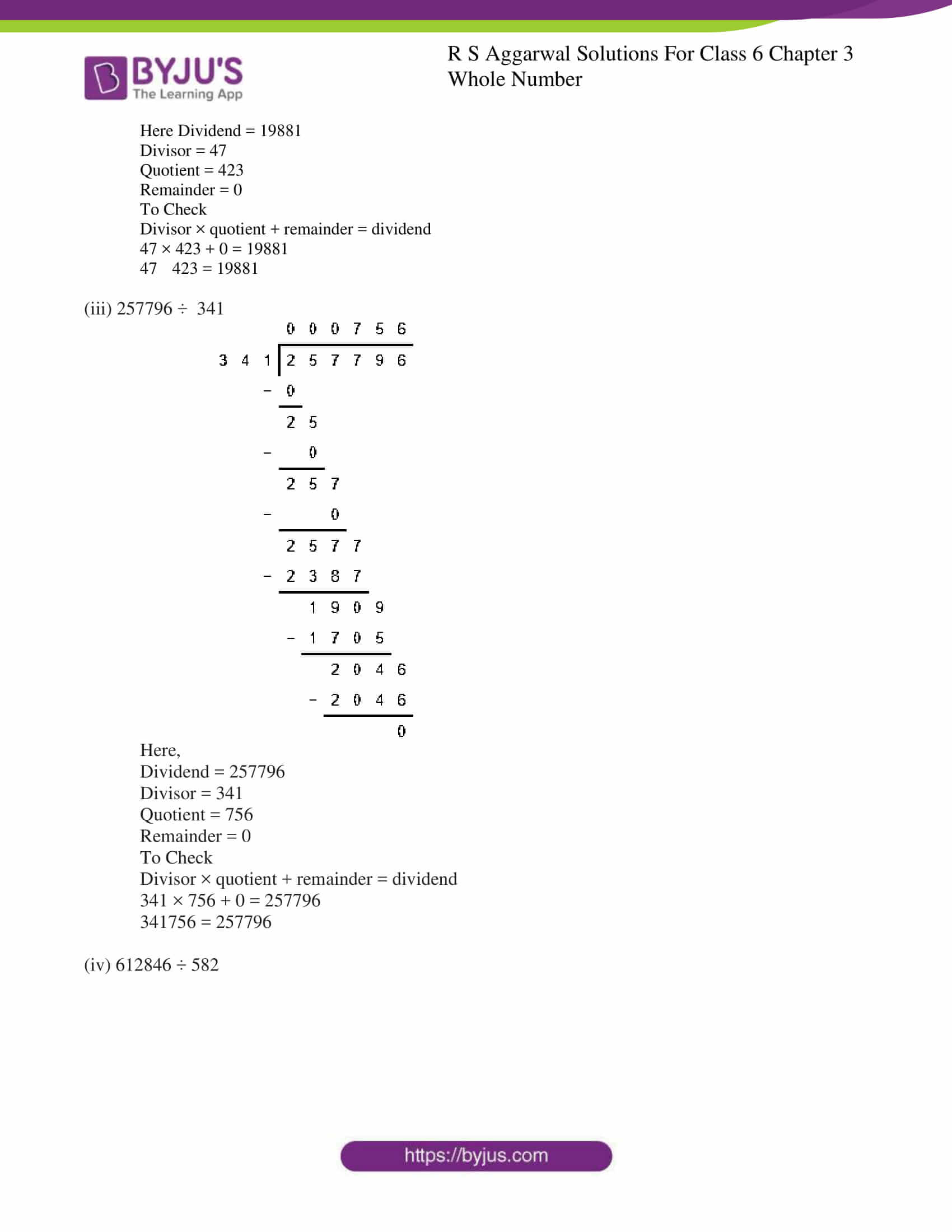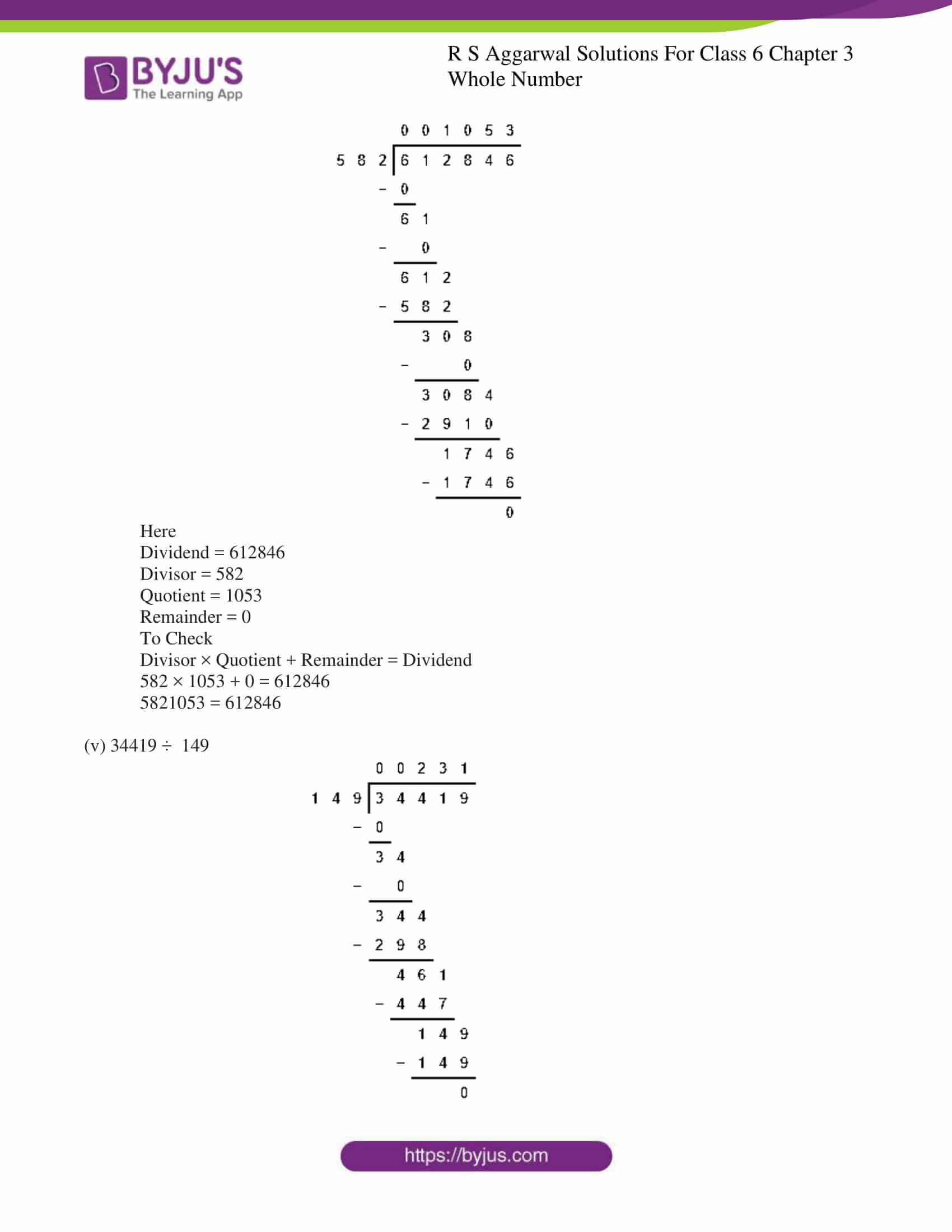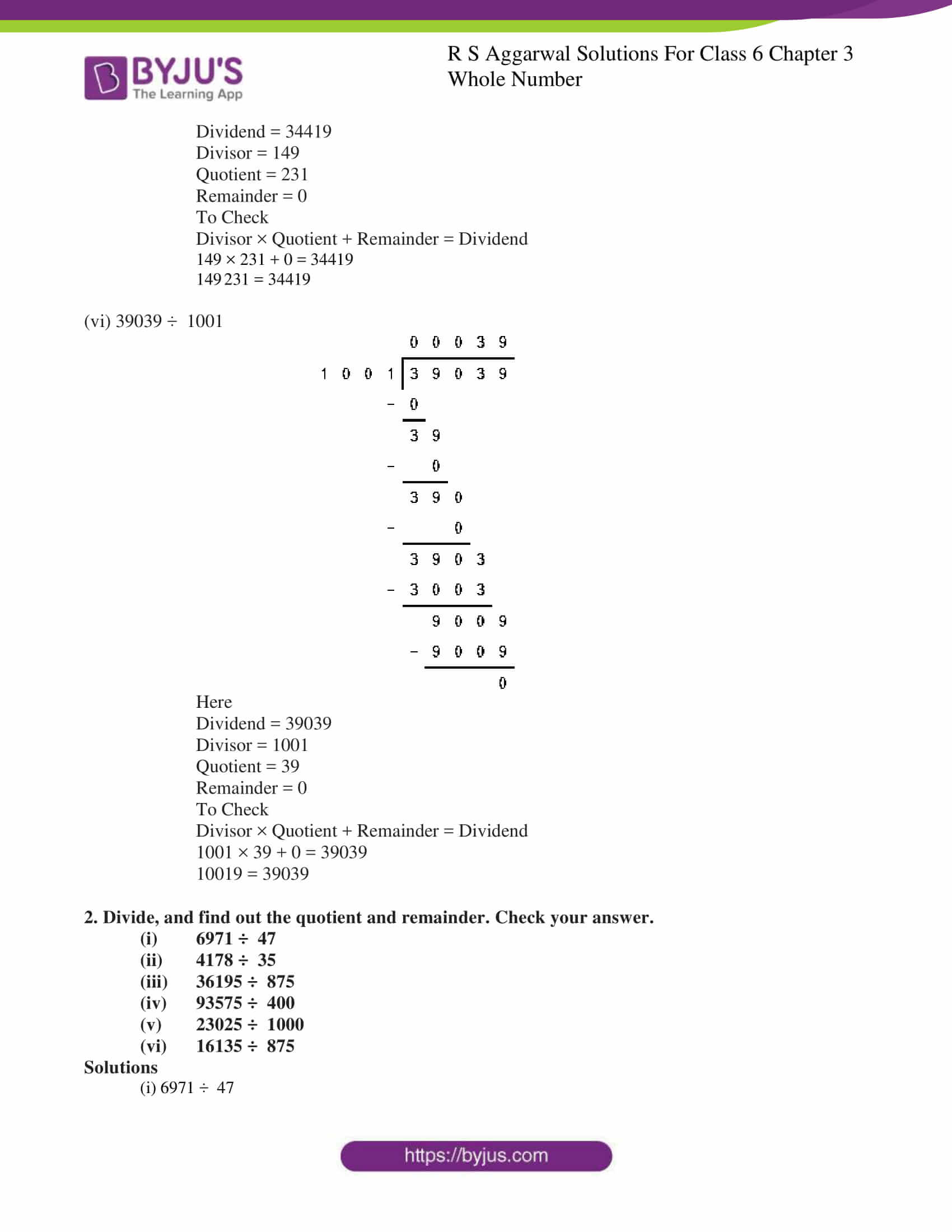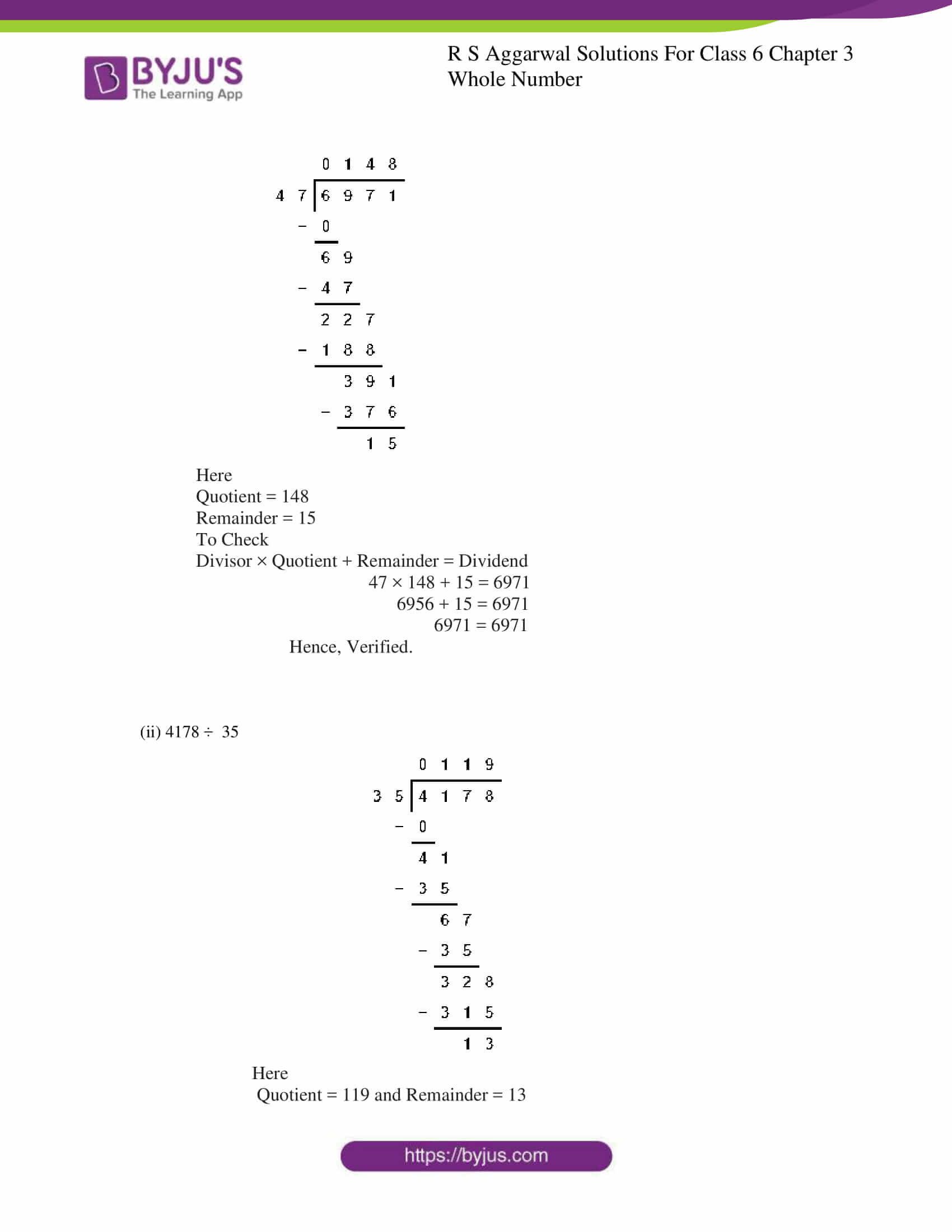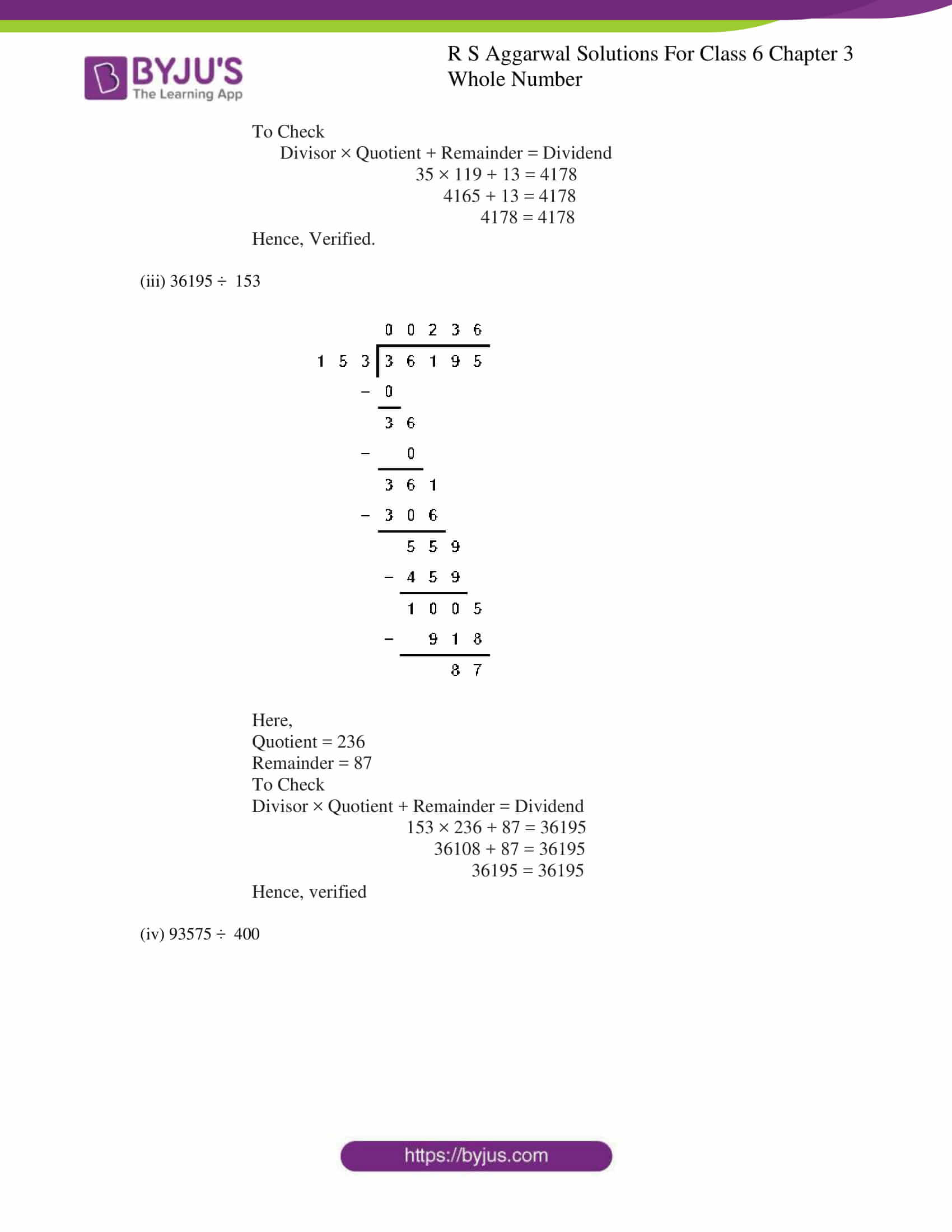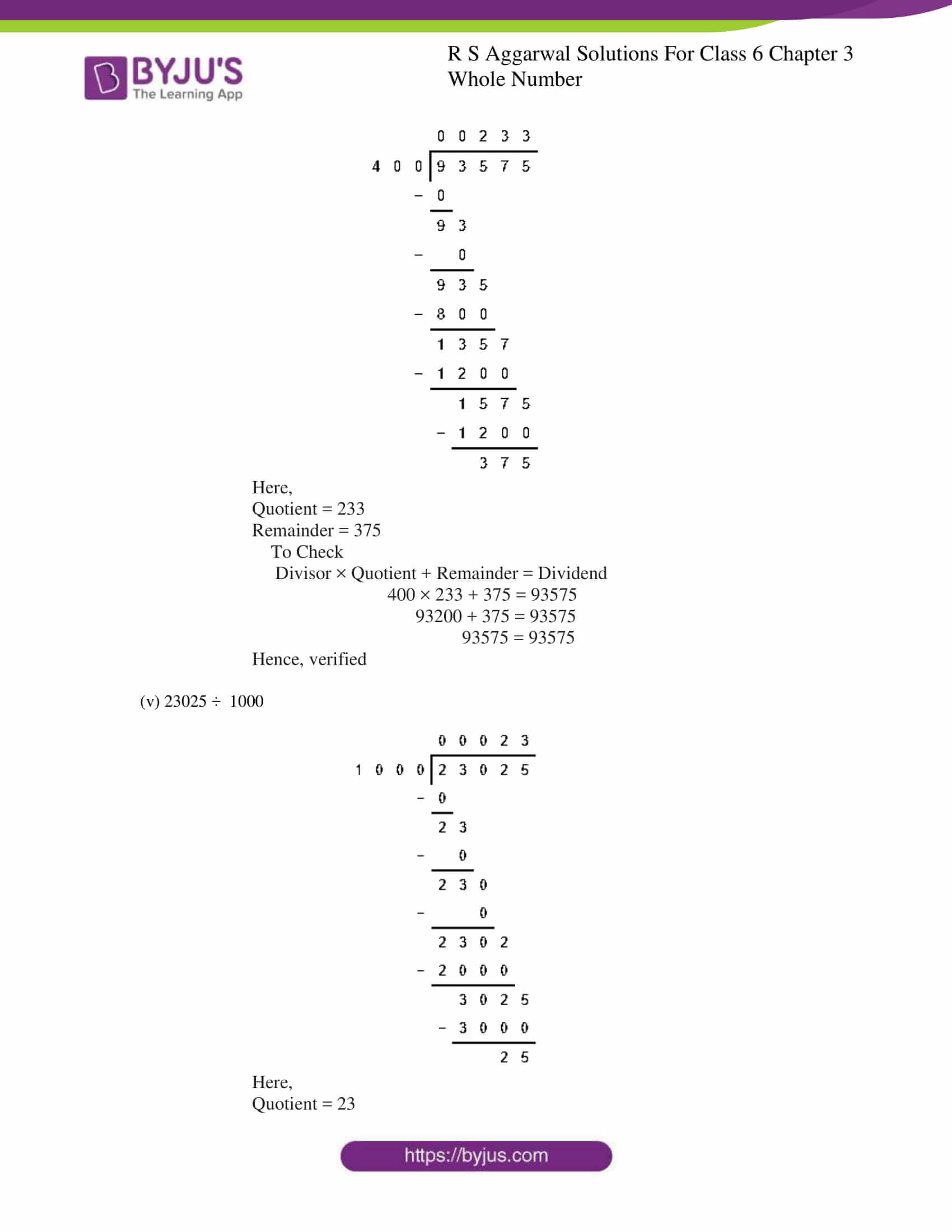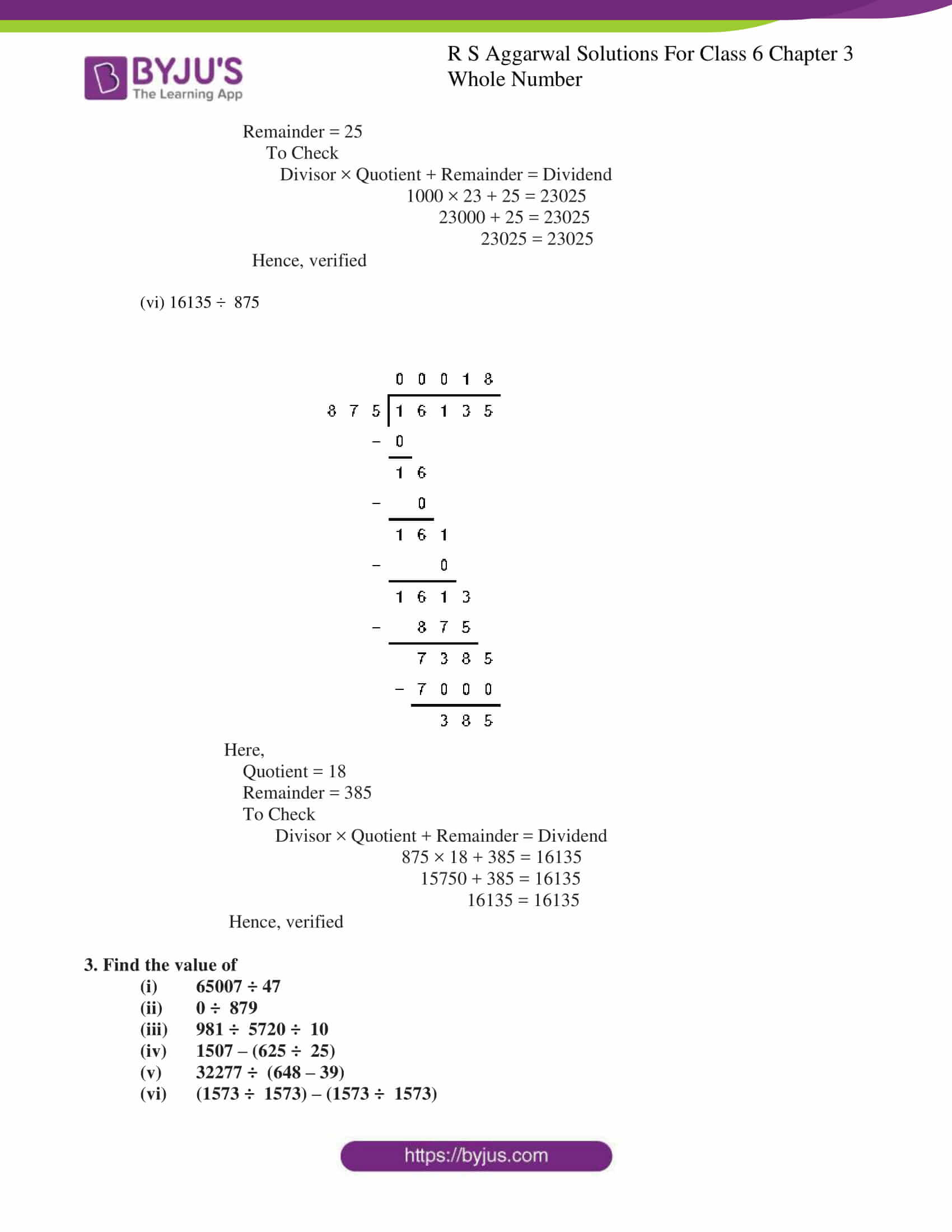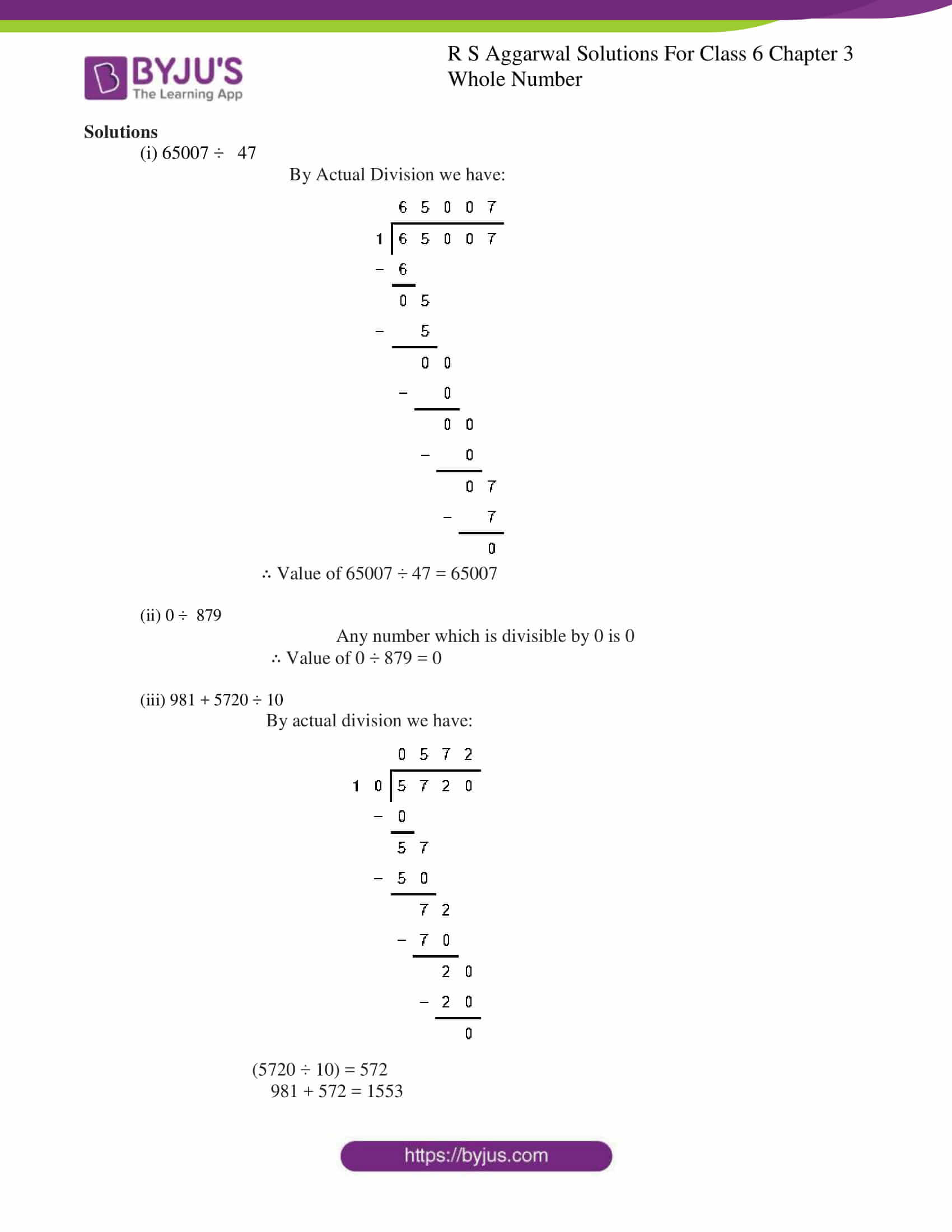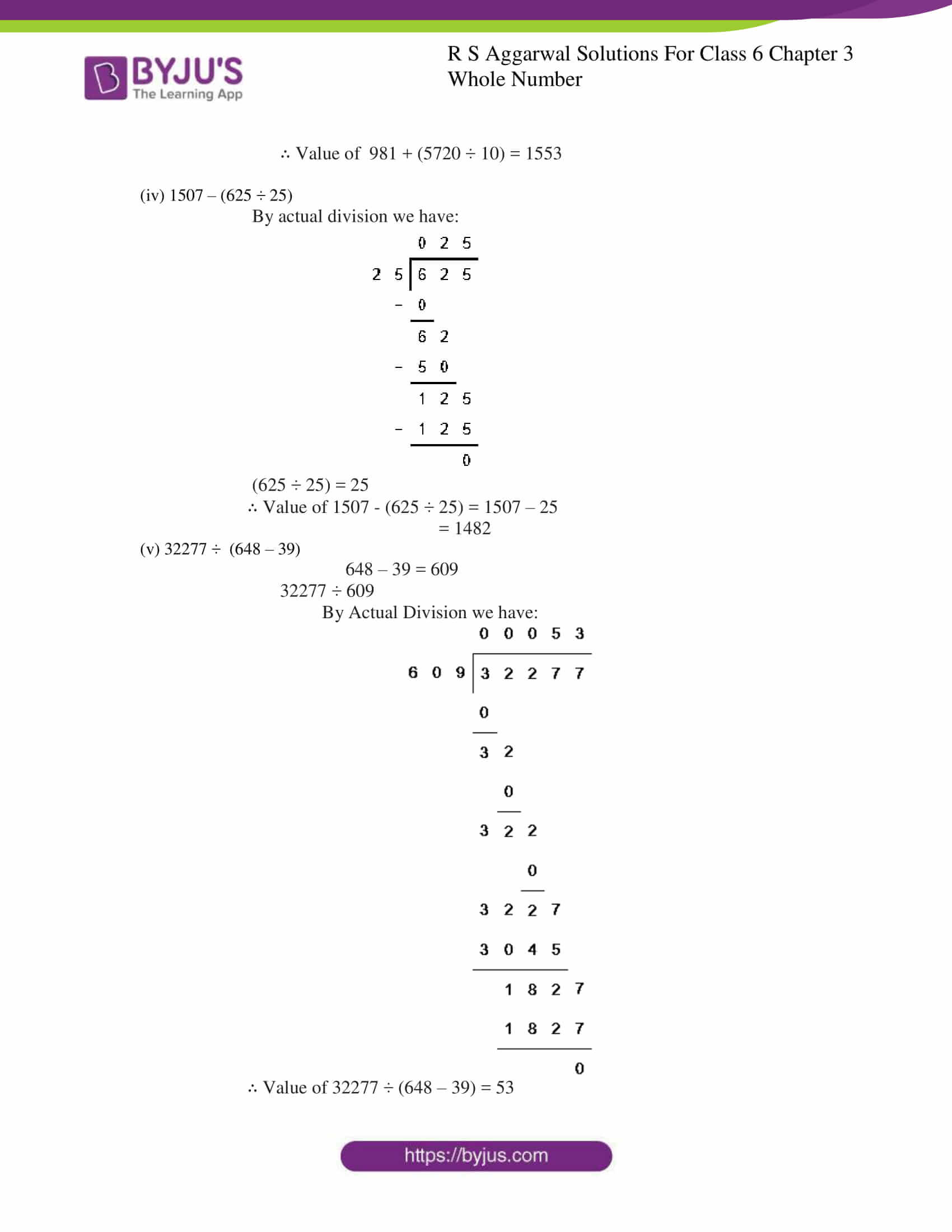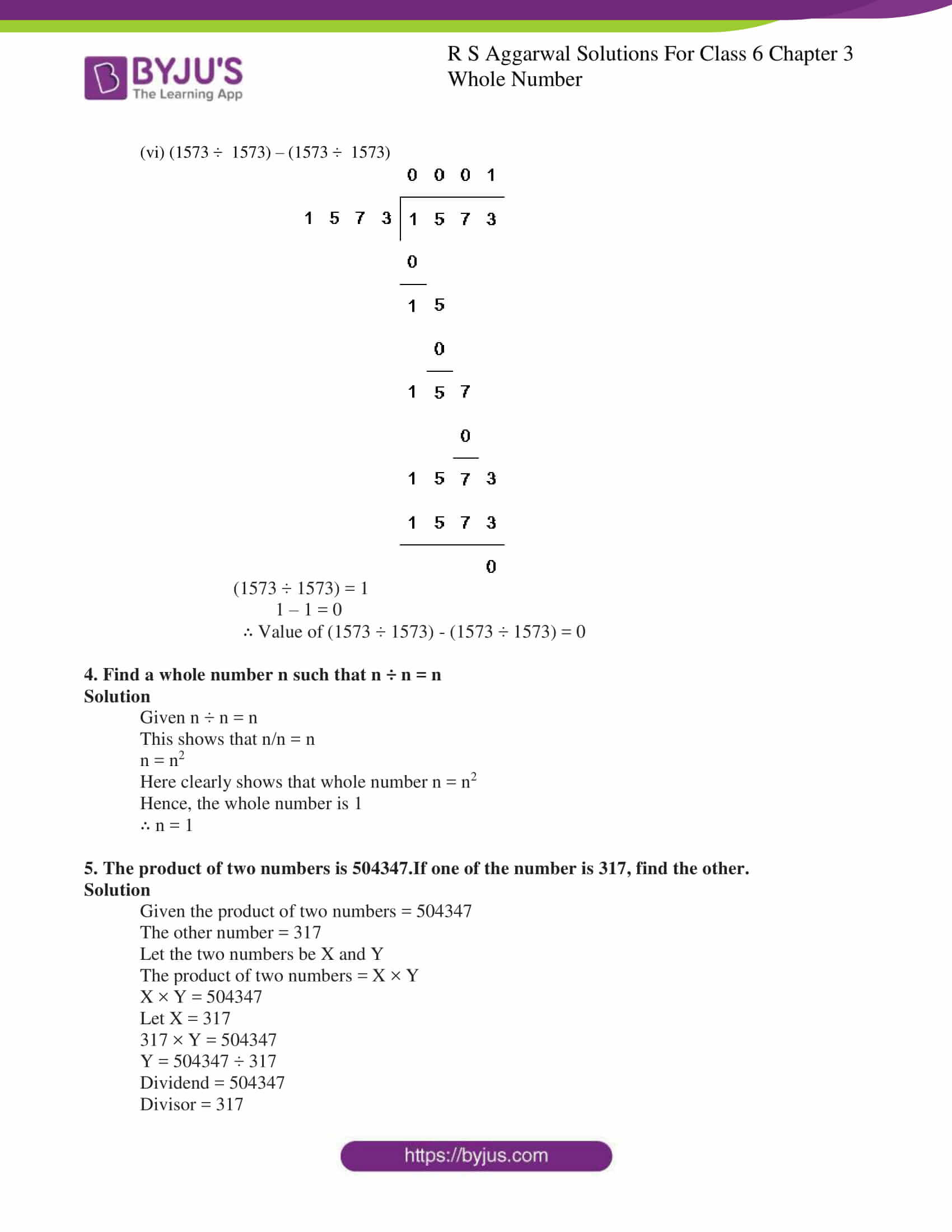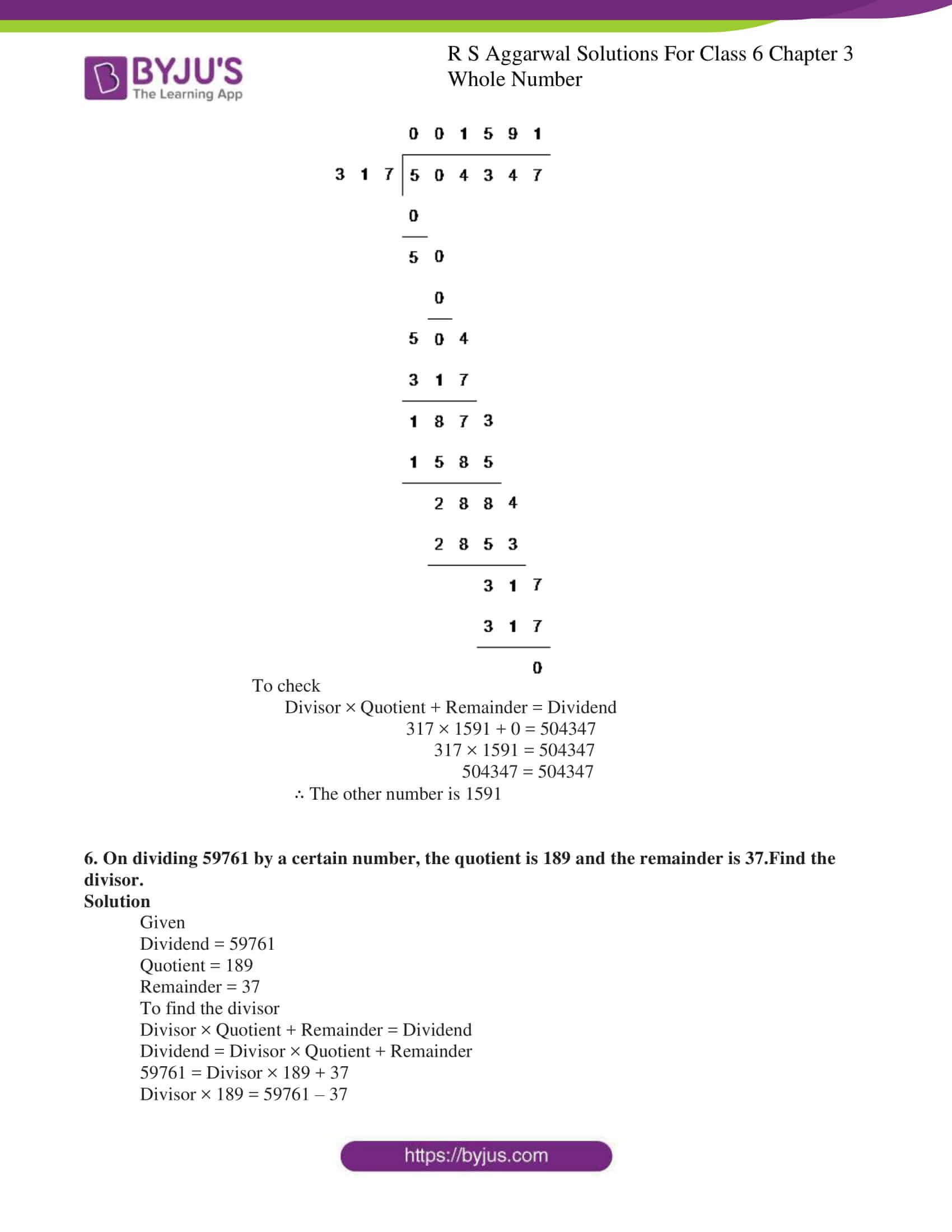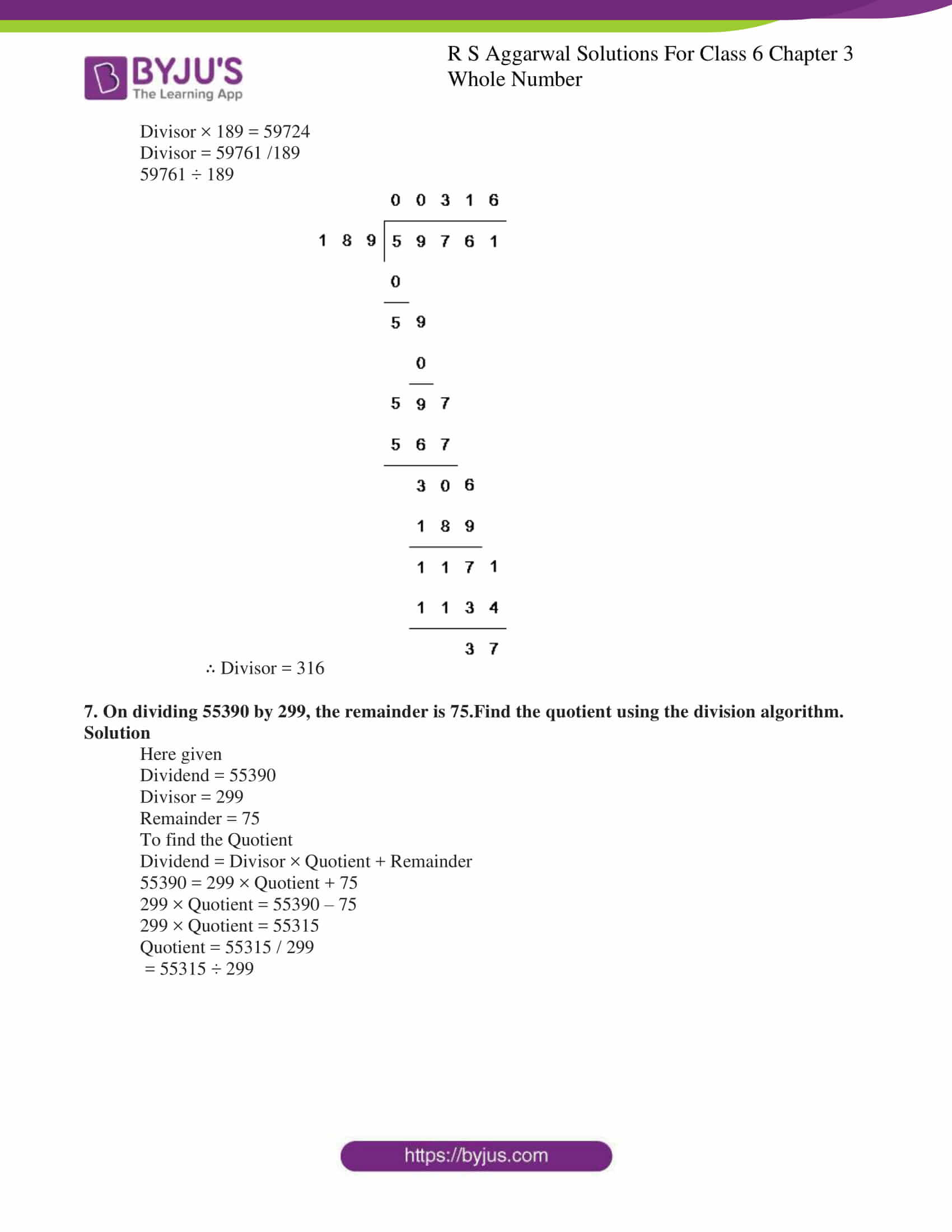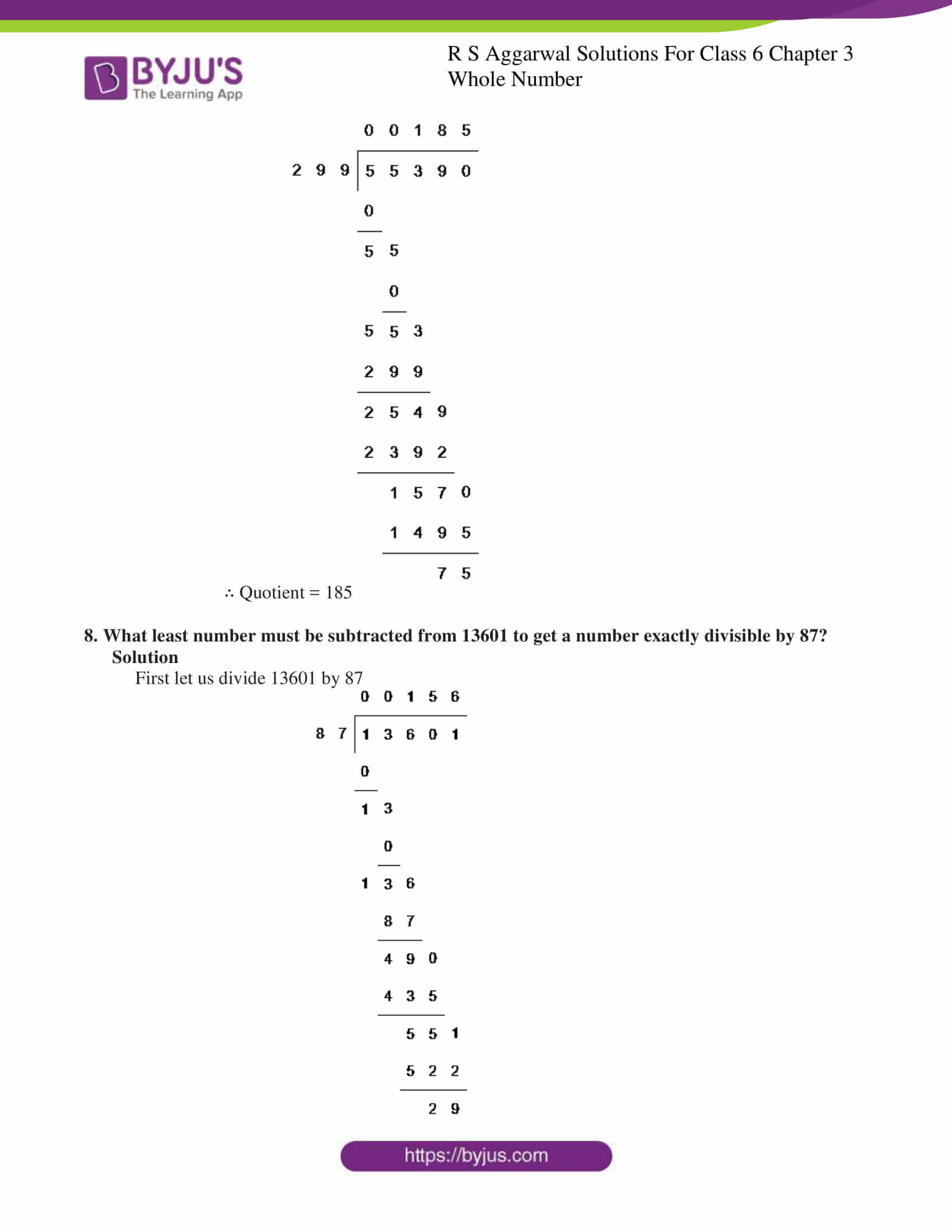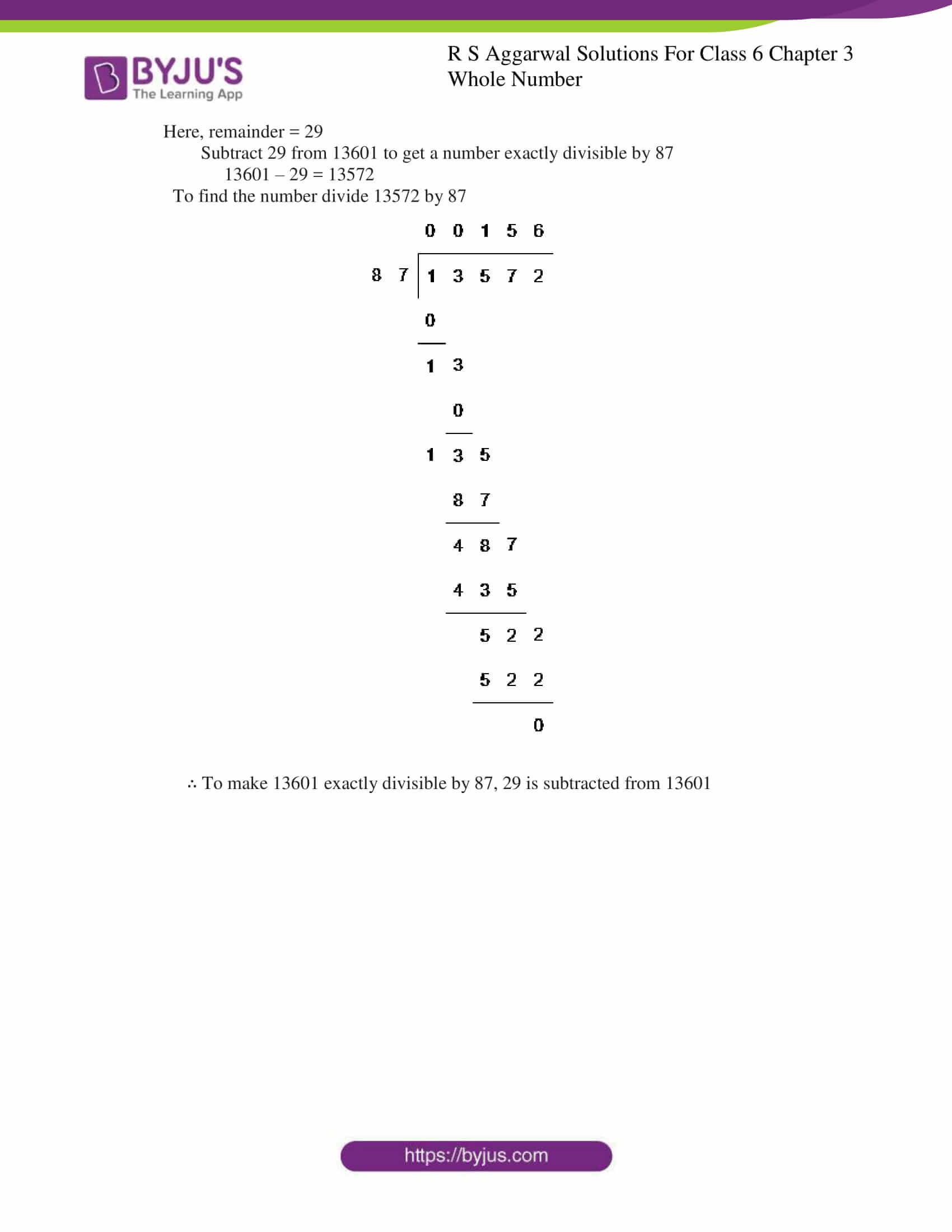### Access answers to Maths RS Aggarwal Solutions for Class 6 Chapter 3 Whole Numbers Exercise 3E

1. Divide and check your answer by the corresponding multiplication in each of the following:

(i) 1936 ÷ 16 (ii) 19881 ÷  47 (iii) 257796 ÷  341 (iv) 6128 ÷  582

(v) 34419 ÷ 149 (vi) 39039 ÷ 1001

Solutions

(i) 1936 ÷ 16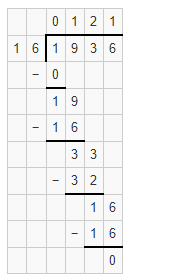Here Dividend = 1936

Divisor = 16

Quotient = 121

Remainder = 0

To check divisor × quotient + remainder = dividend

16 × 121 + 0 = 1936

1. 121 = 1936

(ii) 19881 ÷  47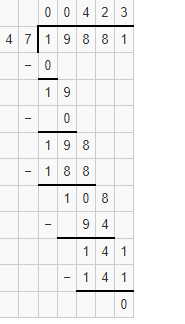Here Dividend = 19881

Divisor = 47

Quotient = 423

Remainder = 0

To Check

Divisor × quotient + remainder = dividend

47 × 423 + 0 = 19881

1. 423 = 19881

(iii) 257796 ÷  341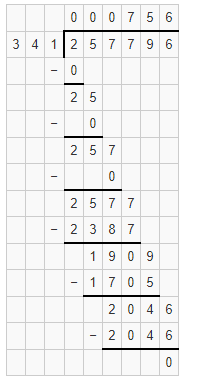Here,

Dividend = 257796

Divisor = 341

Quotient = 756

Remainder = 0

To Check

Divisor × quotient + remainder = dividend

341 × 756 + 0 = 257796

1. 756 = 257796

(iv) 612846 ÷ 582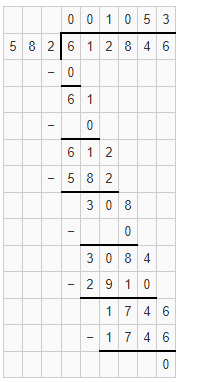Here

Dividend = 612846

Divisor = 582

Quotient = 1053

Remainder = 0

To Check

Divisor × Quotient + Remainder = Dividend

582 × 1053 + 0 = 612846

1. 1053 = 612846

(v) 34419 ÷  149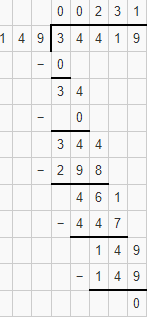Dividend = 34419

Divisor = 149

Quotient = 231

Remainder = 0

To Check

Divisor × Quotient + Remainder = Dividend

149 × 231 + 0 = 34419

1. 231 = 34419

(vi) 39039 ÷  1001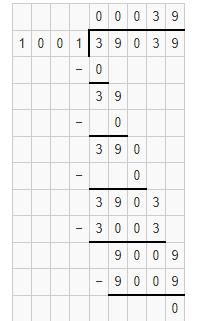Here

Dividend = 39039

Divisor = 1001

Quotient = 39

Remainder = 0

To Check

Divisor × Quotient + Remainder = Dividend

1001 × 39 + 0 = 39039

1. 9 = 39039

2. Divide, and find out the quotient and remainder. Check your answer.

1. 6971 ÷  47
2. 4178 ÷  35
3. 36195 ÷  875
4. 93575 ÷  400
5. 23025 ÷  1000
6. 16135 ÷  875

Solutions

(i) 6971 ÷  47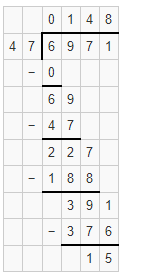Here

Quotient = 148

Remainder = 15

To Check

Divisor × Quotient + Remainder = Dividend

47 × 148 + 15 = 6971

6956 + 15 = 6971

6971 = 6971

Hence, Verified.

(ii) 4178 ÷  35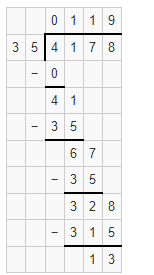Here

Quotient = 119 and Remainder = 13

To Check

Divisor × Quotient + Remainder = Dividend

35 × 119 + 13 = 4178

4165 + 13 = 4178

4178 = 4178

Hence, Verified.

(iii) 36195 ÷  153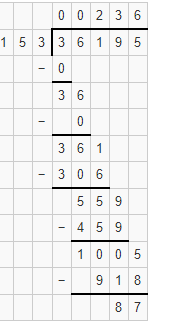Here,

Quotient = 236

Remainder = 87

To Check

Divisor × Quotient + Remainder = Dividend

153 × 236 + 87 = 36195

36108 + 87 = 36195

36195 = 36195

Hence, verified

(iv) 93575 ÷  400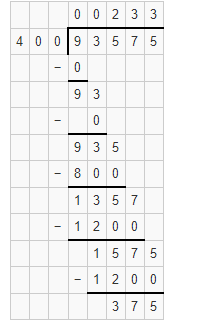Here,

Quotient = 233

Remainder = 375

To Check

Divisor × Quotient + Remainder = Dividend

400 × 233 + 375 = 93575

93200 + 375 = 93575

93575 = 93575

Hence, verified

(v) 23025 ÷  1000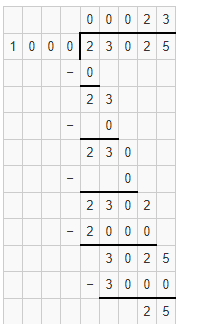Here,

Quotient = 23

Remainder = 25

To Check

Divisor × Quotient + Remainder = Dividend

1000 × 23 + 25 = 23025

23000 + 25 = 23025

23025 = 23025

Hence, verified

(vi) 16135 ÷  875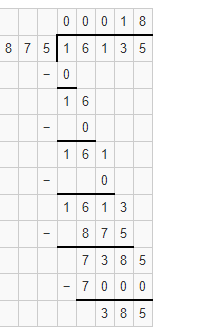Here,

Quotient = 18

Remainder = 385

To Check

Divisor × Quotient + Remainder = Dividend

875 × 18 + 385 = 16135

15750 + 385 = 16135

16135 = 16135

Hence, verified

3. Find the value of

1. 65007 ÷ 47
2. 0 ÷  879
3. 981 ÷  5720 ÷  10
4. 1507 – (625 ÷  25)
5. 32277 ÷  (648 – 39)
6. (1573 ÷  1573) – (1573 ÷  1573)

Solutions

(i) 65007 ÷  47

By Actual Division we have: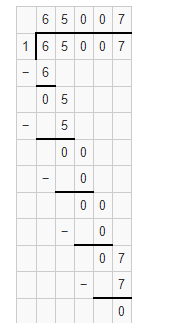∴ Value of 65007 ÷ 47 = 65007

(ii) 0 ÷  879

Any number which is divisible by 0 is 0

∴ Value of 0 ÷ 879 = 0

(iii) 981 + 5720 ÷ 10

By actual division we have: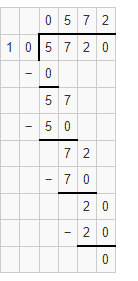(5720 ÷ 10) = 572

981 + 572 = 1553

∴ Value of 981 + (5720 ÷ 10) = 1553

(iv) 1507 – (625 ÷ 25)

By actual division we have: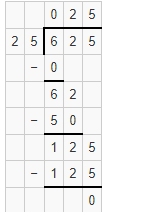(625 ÷ 25) = 25

∴ Value of 1507 – (625 ÷ 25) = 1507 – 25

= 1482

(v) 32277 ÷  (648 – 39)

648 – 39 = 609

32277 ÷ 609

By Actual Division we have: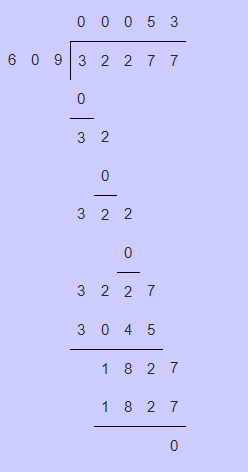∴ Value of 32277 ÷ (648 – 39) = 53

(vi) (1573 ÷  1573) – (1573 ÷  1573)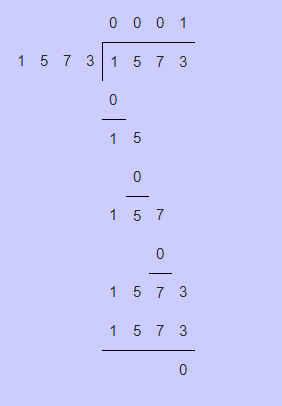(1573 ÷ 1573) = 1

1 – 1 = 0

∴ Value of (1573 ÷ 1573) – (1573 ÷ 1573) = 0

4. Find a whole number n such that n ÷ n = n

Solution

Given n ÷ n = n

This shows that n/n = n

n = n2

Here clearly shows that whole number n = n2

Hence, the whole number is 1

∴ n = 1

5. The product of two numbers is 504347.If one of the number is 317, find the other.

Solution

Given the product of two numbers = 504347

The other number = 317

Let the two numbers be X and Y

The product of two numbers = X × Y

X × Y = 504347

Let X = 317

317 × Y = 504347

Y = 504347 ÷ 317

Dividend = 504347

Divisor = 317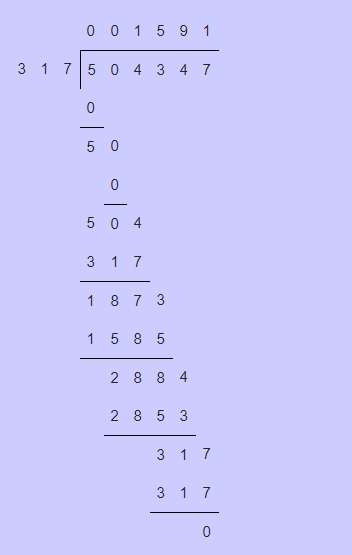To check

Divisor × Quotient + Remainder = Dividend

317 × 1591 + 0 = 504347

317 × 1591 = 504347

504347 = 504347

∴ The other number is 1591

6. On dividing 59761 by a certain number, the quotient is 189 and the remainder is 37.Find the divisor.

Solution

Given

Dividend = 59761

Quotient = 189

Remainder = 37

To find the divisor

Divisor × Quotient + Remainder = Dividend

Dividend = Divisor × Quotient + Remainder

59761 = Divisor × 189 + 37

Divisor × 189 = 59761 – 37

Divisor × 189 = 59724

Divisor = 59761 /189

59761 ÷ 189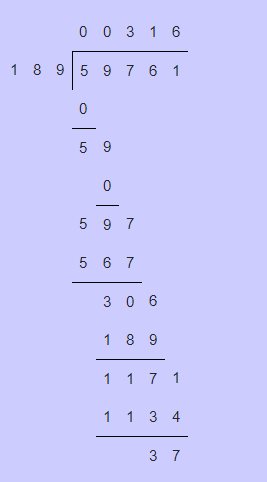∴ Divisor = 316

7. On dividing 55390 by 299, the remainder is 75.Find the quotient using the division algorithm.

Solution

Here given

Dividend = 55390

Divisor = 299

Remainder = 75

To find the Quotient

Dividend = Divisor × Quotient + Remainder

55390 = 299 × Quotient + 75

299 × Quotient = 55390 – 75

299 × Quotient = 55315

Quotient = 55315 / 299

= 55315 ÷ 299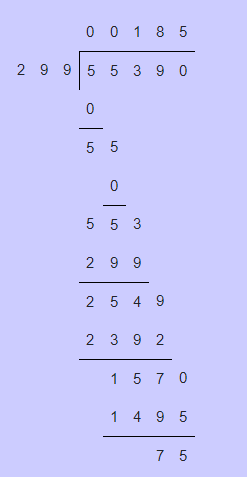∴ Quotient = 185

8. What least number must be subtracted from 13601 to get a number exactly divisible by 87?

Solution

First let us divide 13601 by 87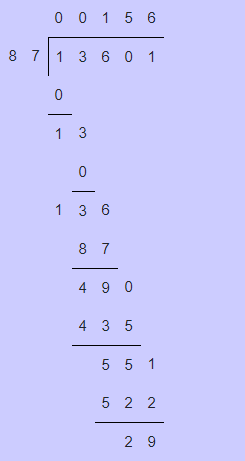Here, remainder = 29

Subtract 29 from 13601 to get a number exactly divisible by 87

13601 – 29 = 13572

To find the number divide 13572 by 87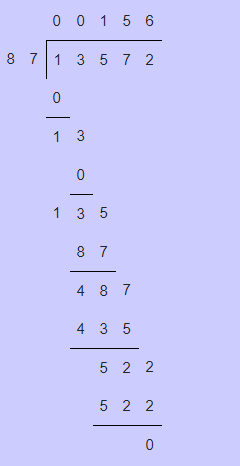∴ To make 13601 exactly divisible by 87, 29 is subtracted from 13601